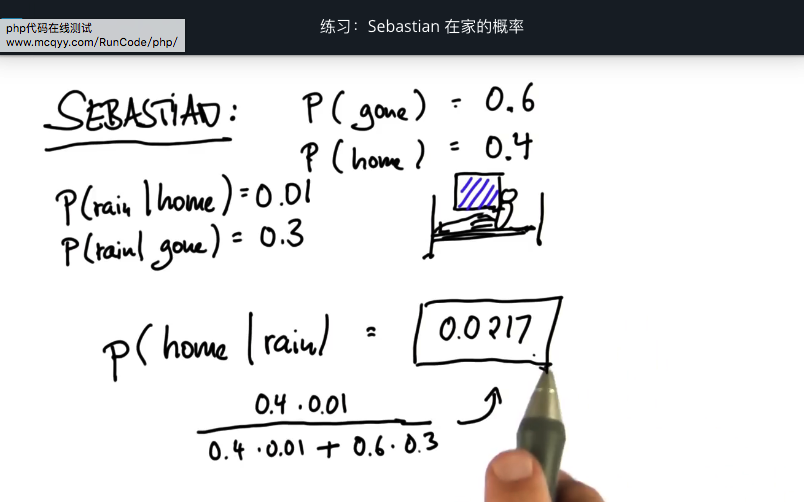# 贝叶斯法则

Bayes Rule 贝叶斯法则

BAYES RULE 贝叶斯法则

## 病理推断

holy grail 概率推理部分

prior probability 先验规则
posterior probability 后验概率

Prior:P(C) = 0.01 = 1%
P(Pos|C) = 0.9 = 90%

posterior: P(C|Pos）= P(C) * P(Pos|C)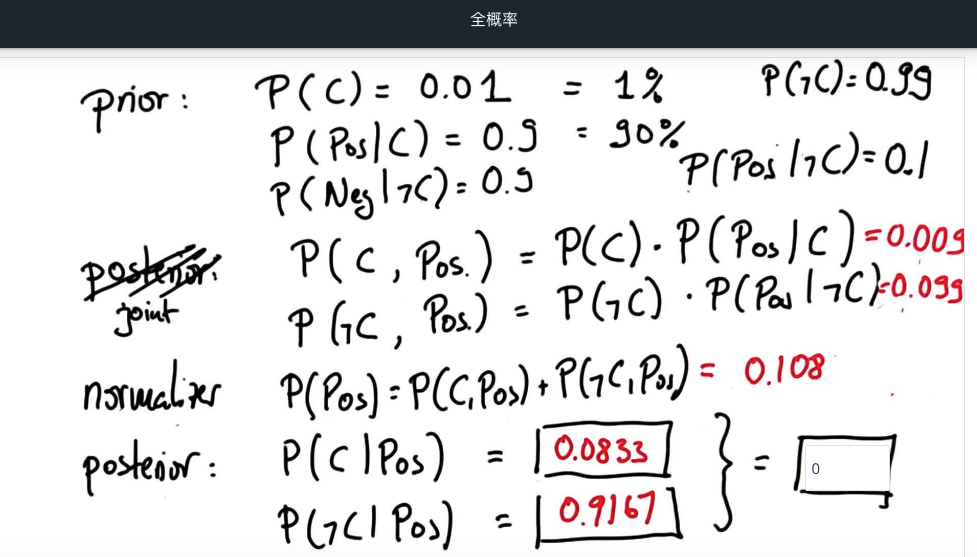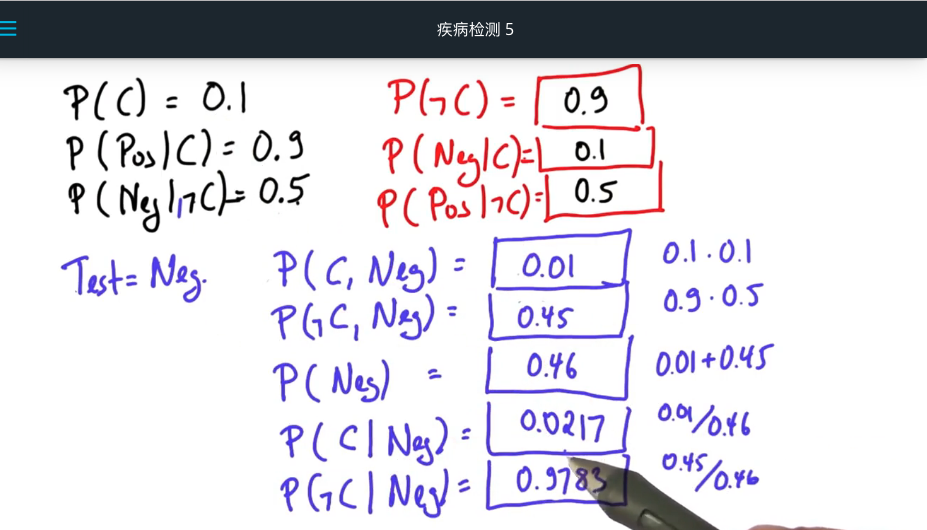## 机器人传感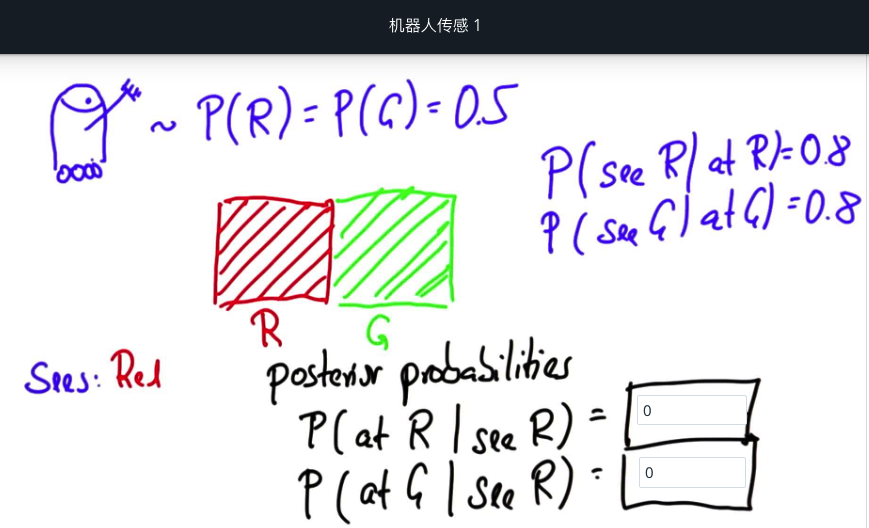P(at red)=0.5
P(at green)=0.5

### 条件概率

P(see red∣at red)=0.8
P(see green∣at green)=0.8

### 后验概率

1. P(at red∣see red)
2. P(at green∣see red)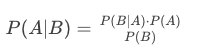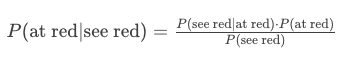### 为什么 P(see red) 是0.5?

#### 方式 2: 数学 （全概率法则）

• 1.当机器人处于红色格子并且其传感器正常工作时。
• 2.当机器人在绿色格子中，其传感器犯了一个错误。 我只需要把这两个概率加起来就可以得到红色的总概率。
P(see red)=P(at red)⋅P(see red∣at red)+P(at green)⋅P(see red∣at green)

P(see red)=0.5⋅0.8+0.5⋅0.2
P(see red)=0.4+0.1
P(see red)=0.5

### 机器人传感8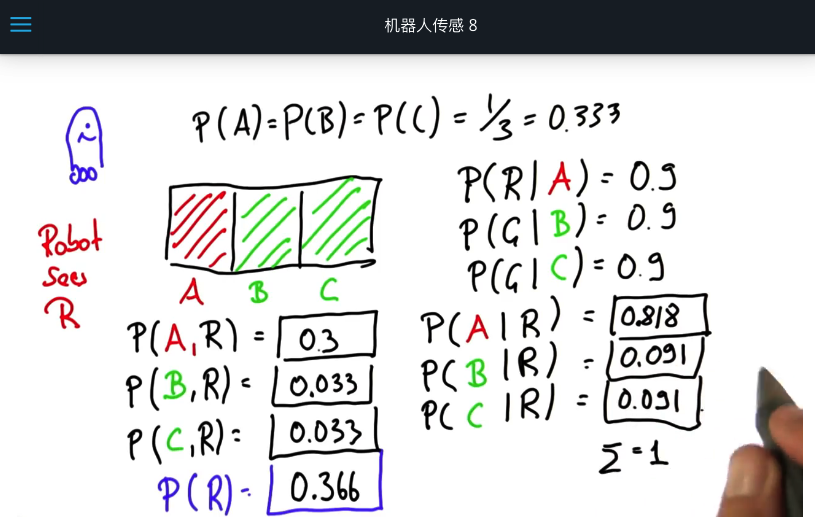### 在家的概率

Sebastian在家的概率为 0.4，在外边的概率为 0.6，在家下雨的概率为 0.01，在外边下雨的概率为0.3，